Next: 4-Dimensional Quantum Gravity Up: Planck Previous: 3-Dimensional Quantum Gravity

# 5. Higher-Dimensional Algebra

One of the most remarkable accomplishments of the early 20th century was to formalize all of mathematics in terms of a language with a deliberately impoverished vocabulary: the language of set theory. In Zermelo-Fraenkel set theory, everything is a set, the only fundamental relationships between sets are membership and equality, and two sets are equal if and only if they have the same elements. If in Zermelo-Fraenkel set theory you ask what sort of thing is the number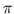, the relationship 'less than', or the exponential function, the answer is always the same: a set! Of course one must bend over backwards to think of such varied entities as sets, so this formalization may seem almost deliberately perverse. However, it represents the culmination of a worldview in which things are regarded as more fundamental than processes or relationships.

More recently, mathematicians have developed a somewhat more flexible language, the language of category theory. Category theory is an attempt to put processes and relationships on an equal status with things. A category consists of a collection of 'objects', and for each pair of objects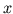and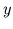, a collection of 'morphisms' fromto. We write a morphism fromtoas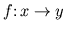. We demand that for any morphismsand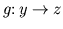, we can 'compose' them to obtain a morphism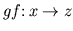. We also demand that composition be associative. Finally, we demand that for any objectthere be a morphism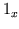, called the 'identity' of, such that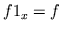for any morphismand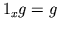for any morphism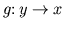.

Perhaps the most familiar example of a category is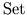. Here the objects are sets and the morphisms are functions between sets. However, there are many other examples. Fundamental to quantum theory is the category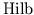. Here the objects are complex Hilbert spaces and the morphisms are linear operators between Hilbert spaces. In Section 3 we also met a category important in differential topology, the category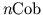. Here the objects are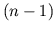-dimensional manifolds and the morphisms are cobordisms between such manifolds. Note that in this example, the morphisms are not functions! Nonetheless we can still think of them as 'processes' going from one object to another.

An important part of learning category theory is breaking certain habits one may have acquired from set theory. For example, in category theory one must resist the temptation to 'peek into the objects'. Traditionally, the first thing one asks about a set is: what are its elements? A set is like a container, and the contents of this container are the most interesting thing about it. But in category theory, an object need not have 'elements' or any sort of internal structure. Even if it does, this is not what really matters! What really matters about an object is its morphisms to and from other objects. Thus category theory encourages a relational worldview in which things are described, not in terms of their constituents, but by their relationships to other things.

Category theory also downplays the importance of equality between objects. Given two elements of a set, the first thing one asks about them is: are they equal? But for objects in a category, we should ask instead whether they are isomorphic. Technically, the objectsandare said to be 'isomorphic' if there is an morphismthat has an 'inverse': a morphism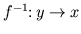for which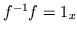and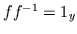. A morphism with an inverse is called an 'isomorphism'. An isomorphism between two objects lets us turn any morphism to or from one of them into a morphism to or from the other in a reversible sort of way. Since what matters about objects are their morphisms to and from other objects, specifying an isomorphism between two objects lets us treat them as 'the same' for all practical purposes.

Categories can be regarded as higher-dimensional analogs of sets. As shown in Figure 5, we may visualize a set as a bunch of points, namely its elements. Similarly, we may visualize a category as a bunch of points corresponding to its objects, together with a bunch of 1-dimensional arrows corresponding to its morphisms. (For simplicity, I have not drawn the identity morphisms in this figure.)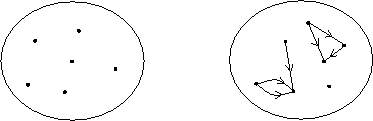We may use the analogy between sets and categories to 'categorify' almost any set-theoretic concept, obtaining a category-theoretic counterpart . For example, just as there are functions between sets, there are 'functors' between categories. A function from one set to another sends each element of the first to an element of the second. Similarly, a functor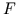from one category to another sends each objectof the first to an object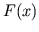of the second, and also sends each morphismof the first to a morphism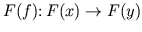of the second. In addition, functors are required to preserve composition and identities: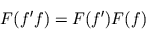and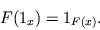Functors are important because they allow us to apply the relational worldview discussed above, not just to objects in a given category, but to categories themselves. Ultimately what matters about a category is not its 'contents' -- its objects and morphisms -- but its functors to and from other categories!

 SET THEORY CATEGORY THEORY elements objects equations between elements isomorphisms between objects sets categories functions between sets functors between categories equations between functions natural isomorphisms between functors

Table 2. Analogy between set theory and category theory

We summarize the analogy between set theory and category theory in Table 2. In addition to the terms already discussed there is a concept of 'natural isomorphism' between functors. This is the correct analog of an equation between functions, but we will not need it here -- I include it just for the sake of completeness.

The full impact of category-theoretic thinking has taken a while to be felt. Categories were invented in the 1940s by Eilenberg and Mac Lane for the purpose of clarifying relationships between algebra and topology. As time passed they became increasingly recognized as a powerful tool for exploiting analogies throughout mathematics . In the early 1960s they led to revolutionary -- and still controversial -- developments in mathematical logic . It gradually became clear that category theory was a part of a deeper subject, 'higher-dimensional algebra', in which the concept of a category is generalized to that of an '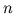-category'. But only by the 1990s did the real importance of categories for physics become evident, with the discovery that higher-dimensional algebra is the perfect language for topological quantum field theory [14,20].

Why are categories important in topological quantum field theory? The most obvious answer is that a TQFT is a functor. Recall from Section 3 that a TQFT maps each manifold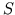representing space to a Hilbert space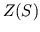and each cobordismrepresenting spacetime to an operator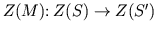, in such a way that composition and identities are preserved. We may summarize all this by saying that a TQFT is a functor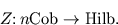In short, category theory makes the analogy in Table 1 completely precise. In terms of this analogy, many somewhat mysterious aspects of quantum theory correspond to easily understood facts about spacetime! For example, the noncommutativity of operators in quantum theory corresponds to the noncommutativity of composing cobordisms. Similarly, the all-important 'adjoint' operation in quantum theory, which turns an operator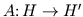into an operator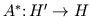, corresponds to the operation of reversing the roles of past and future in a cobordism, obtaining a cobordism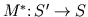.

But the role of category theory goes far beyond this. The real surprise comes when one examines the details of specific TQFTs. In Section 4 I sketched the construction of 3-dimensional quantum gravity, but I left out the recipe for computing amplitudes for spacetime geometries. Thus the most interesting features of the whole business were left as unexplained 'miracles': the background-independence of the Hilbert spacesand operators, and the fact that they satisfy Atiyah's axioms for a TQFT. In fact, the recipe for amplitudes and the verification of these facts make heavy use of category theory. The same is true for all other theories for which Atiyah's axioms have been verified. For some strange reason, it seems that category theory is precisely suited to explaining what makes a TQFT tick.

For the last 10 years or so, various researchers have been trying to understand this more deeply. Much remains mysterious, but it now seems that TQFTs are intimately related to category theory because of special properties of the category. Whileis defined using concepts from differential topology, a great deal of evidence suggests that it admits a simple description in terms of '-categories'.

I have already alluded to the concept of 'categorification' -- the process of replacing sets by categories, functions by functors and so on, as indicated in Table 2. The concept of '-category' is obtained from the concept of 'set' by categorifying ittimes! An-category has objects, morphisms between objects, 2-morphisms between morphisms, and so on up to-morphisms, together with various composition operations satisfying various reasonable laws . Increasing the value ofallows an ever more nuanced treatment of the notion of 'sameness'. A 0-category is just a set, and in a set the elements are simply equal or unequal. A 1-category is a category, and in this context we may speak not only of equal but also of isomorphic objects. Unfortunately, this careful distinction between equality and isomorphism breaks down when we study the morphisms. Morphisms in a category are either the same or different; there is no concept of isomorphic morphisms. In a 2-category this is remedied by introducing 2-morphisms between morphisms. Unfortunately, in a 2-category we cannot speak of isomorphic 2-morphisms. To remedy this we must introduce the notion of 3-category, and so on.

We may visualize the objects of an-category as points, the morphisms as arrows going between these points, the 2-morphisms as 2-dimensional surfaces going between these arrows, and so on. There is thus a natural link between-categories and-dimensional topology. Indeed, one reason why-categories are a bit formidable is that calculations with them are most naturally done using-dimensional diagrams. But this link between-categories and-dimensional topology is precisely why there may be a nice description ofin the language of-categories.

Dolan and I have proposed such a description, which we call the 'cobordism hypothesis' . Much work remains to be done to make this hypothesis precise and prove or disprove it. Proving it would lay the groundwork for understanding topological quantum field theories in a systematic way. But beyond this, it would help us towards a purely algebraic understanding of 'space' and 'spacetime' -- which is precisely what we need to marry them to the quantum-mechanical notions of 'state' and 'process'.

Next: 4-Dimensional Quantum Gravity Up: Planck Previous: 3-Dimensional Quantum Gravity